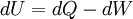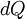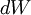# First Law of Thermodynamics

The First Law of Thermodynamics states that the increase in internal energy of a closed system equals the amount of heat energy added minus the work performed by the system.

Mathematically, this is described as follows:whereis the infinitesimal increase in the internal energyis the infinitesimal amount of heat added to the systemis the infinitesimal amount of work performed done by the system

A ramification of this is the Principle of conservation of energy; the amount of energy in the closed system of the universe remains constant. This can be combined with the principle of Mass-Energy Equivalence to demonstrate that the amount of mass in the universe is constant.

Note that if no heat is added, then the maximum amount of work that can be performed by the system is equal to its internal energy. This prevents the existence of a type of perpetual motion machine. More generally, if no energy is added to a system, the maximum amount of work the system can perform is equal to its initial energy. This may be in several forms, such as kinetic energy or potential energy.

## The 1st and 2nd law of thermodynamics and the universe having a beginning

According to Ohio State University professor Patrick Woodward, the First Law of Thermodynamics "simply states that energy can be neither created nor destroyed (conservation of energy)."

The Christian apologetics website Why believe in God? declares about the Second Law of Thermodynamics:

 “ The second law of thermodynamics, or the law of increased entropy, says that over time, everything breaks down and tends towards disorder - entropy! Entropy is the amount of UNusable energy in any systems; that system could be the earth's environment or the universe itself. The more entropy there is, the more disorganisation and chaos. Therefore, if no outside force is adding energy to an isolated system to help renew it, it will eventually burn out (heat death). This can be applied to a sun as well as a cup of tea - left to themselves, both will grow cold. You can heat up a cold tea, you cannot heat up a cold sun. NOTE: when a hot tea in an air tight room goes cold (loses all it's energy) not only do we NOT expect the process to reverse by natural causes (ie. the tea will get hot again), but both room temp and tea temp will be equal. Keep that in mind as you read the next paragraph. Look at it like this, because the energy in the universe is finite and no new energy is being added to it (1st law), and because the energy is being used up (2nd law), the universe cannot be infinite. If our universe was infinite but was using up a finite supply of energy, it would have suffered 'heat death' a long time ago! If the universe was infinite all radioactive atoms would have decayed and the universe would be the same temperature with no hot spots, no bright burning stars. Since this is not true, the universe must have begun a finite time ago. ”

Thus, the First Law of Thermodynamics and the Second Law of Thermodynamics point to the universe having a beginning.